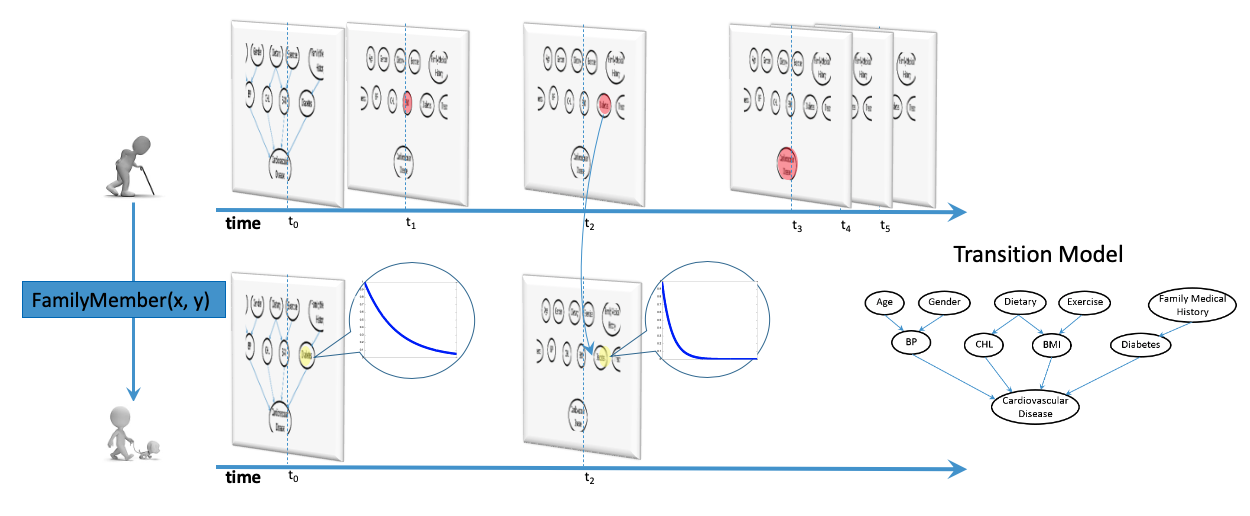# Sequence Data Mining

Relational Continuous Time Bayesian Network

In this project, we consider the problem of predicting sequential events by learning from relational trajectories with dinstinct temporal resolutions.

Most of the real-world events occur at their distinct paces, some events happen more frequently than others. However, for most of the dynamic models such as HMM, DBNs, or RNN, they model such dynamic process with constant interval between the time slices. So, in order to avoid missing any events along the trajectories, they need to model the system at the shortest possible interval. This leads to the increased computational cost when performing inference over time. Continuous Time Bayesian Networks (CTBN), on the other hand, models the time directly by assuming the probability for certain event to happen is an exponential function of time. So it can answer queries at any time point.

Moreover, relations are widely existing among the trajectories of features from different objects. Take the following network as an example. The chance of getting type 2 diabetes increases with age, so its transition probability can be modeled with an exponential function of time. it is also a hereditary disease, so the transition likelihood of a person’s diabetes status depends on the trajectory of his family members’ diabetes status. If a family member of the target object has been diagnosis with diabetes at time 2, then our belief in the transition probability of this individual’s diabetes status would have larger transition parameter from this time point on. The solution for the structured data is the statistical relational models.We developed the first relational representation for Continuous-Time modeling. It features a nonparametric learning method that allows for efficiently learning the complex dependencies and their strengths simultaneously from relational trajectories data.

# How to use this package?